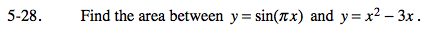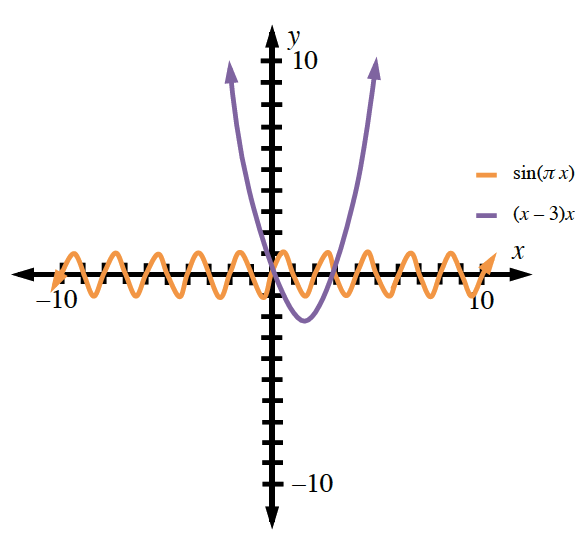### Home > CALC > Chapter 5 > Lesson 5.1.3 > Problem5-28

5-28.

Find the area between y = sin(πx) and y = x2 − 3x. Homework Help ✎Use your calculator to find the points of intersection between the two graphs.
Then determine which graph is on top (has higher y-values) and which is below.5.137 units2